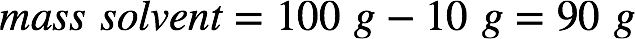# Problem: An aqueous solution is 10.% glucose by mass (d = 1.039 g/mL at 20°C). Calculate its freezing point, boiling point at 1 atm, and osmotic pressure.

🤓 Based on our data, we think this question is relevant for Professor Evans' class at QC CUNY .

###### FREE Expert Solution

The mass percent of a solution is given by:In a 10% by mass glucose (C6H12O6) solution, this means there are 10 g of glucose for every 100 g solution. From this, we can calculate the mass of water (the solvent):We need to find the molality of the solution since we'll use it to calculate the freezing point depression and boiling point elevation. Recall that molality is:The molar mass of glucose is 6(12.01 g/mol C) + 12(1.01 g/mol H) + 6(16.00 g/mol O) = 180.18 g/mol. Calculating the molality of the glucose solution:###### Problem Details

An aqueous solution is 10.% glucose by mass (d = 1.039 g/mL at 20°C). Calculate its freezing point, boiling point at 1 atm, and osmotic pressure.